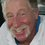# Thoughts on Solution to Spherical Liquid Planet and Pressure problem

Imagine the atoms in the planet as bowling balls (also basically not compressible). Would you rather be under 1, 10, or 100 bowling balls, that is, nearer the surface or nearer the center? Most people would prefer to be under less weight/mass, because the weight (pressure) on them would be less; here, weight is the same as pressure. (The question did not mention temperature, so we can take a temperature as close to 0 Kelvin as we wish, which makes the analogy of the liquid's atoms to a bowling ball, basically not moving, more apt, and removes other possible causes of pressure.) Although gravity in a sphere acts as if all mass were concentrated at the center, because gravitational attraction in all other directions than radially tend to cancel out, the mass actually remains distributed evenly throughout the sphere in this problem, so there is mass/weight to be on top of you if you are within the sphere; you'd prefer as little as possible (less pressure). So intuitively, the pressure is least near the surface and greater at the center. The apparent concentration of all mass at the center means that the gravitational force, which decreases by the square of distance from that point, is greatest nearer the center.Note by Jed Somit
2 years, 10 months ago

This discussion board is a place to discuss our Daily Challenges and the math and science related to those challenges. Explanations are more than just a solution — they should explain the steps and thinking strategies that you used to obtain the solution. Comments should further the discussion of math and science.

When posting on Brilliant:

• Use the emojis to react to an explanation, whether you're congratulating a job well done , or just really confused .
• Ask specific questions about the challenge or the steps in somebody's explanation. Well-posed questions can add a lot to the discussion, but posting "I don't understand!" doesn't help anyone.
• Try to contribute something new to the discussion, whether it is an extension, generalization or other idea related to the challenge.

MarkdownAppears as
*italics* or _italics_ italics
**bold** or __bold__ bold
- bulleted- list
• bulleted
• list
1. numbered2. list
1. numbered
2. list
Note: you must add a full line of space before and after lists for them to show up correctly
paragraph 1paragraph 2

paragraph 1

paragraph 2

[example link](https://brilliant.org)example link
> This is a quote
This is a quote
    # I indented these lines
# 4 spaces, and now they show
# up as a code block.

print "hello world"
# I indented these lines
# 4 spaces, and now they show
# up as a code block.

print "hello world"
MathAppears as
Remember to wrap math in $$ ... $$ or $ ... $ to ensure proper formatting.
2 \times 3 $2 \times 3$
2^{34} $2^{34}$
a_{i-1} $a_{i-1}$
\frac{2}{3} $\frac{2}{3}$
\sqrt{2} $\sqrt{2}$
\sum_{i=1}^3 $\sum_{i=1}^3$
\sin \theta $\sin \theta$
\boxed{123} $\boxed{123}$